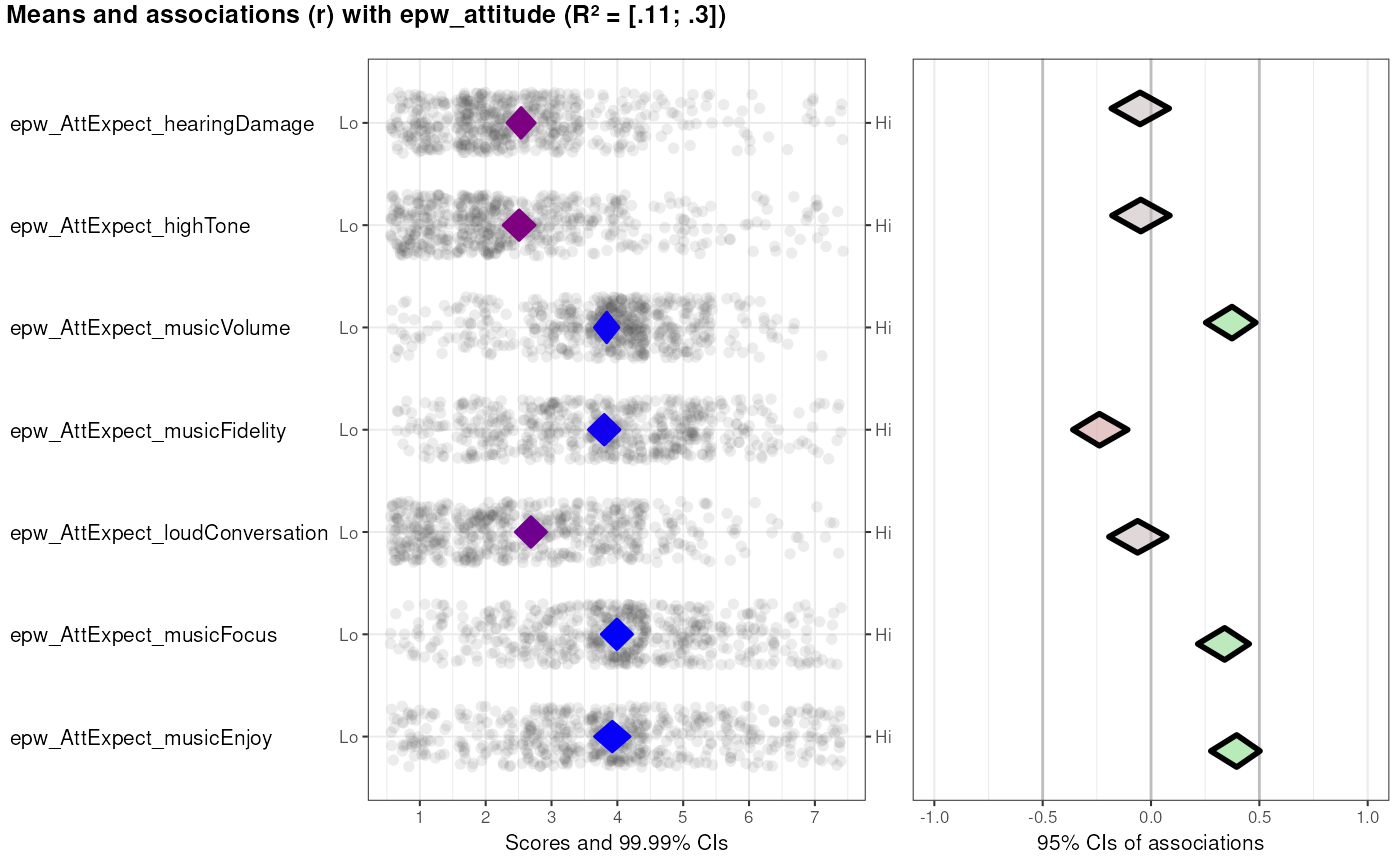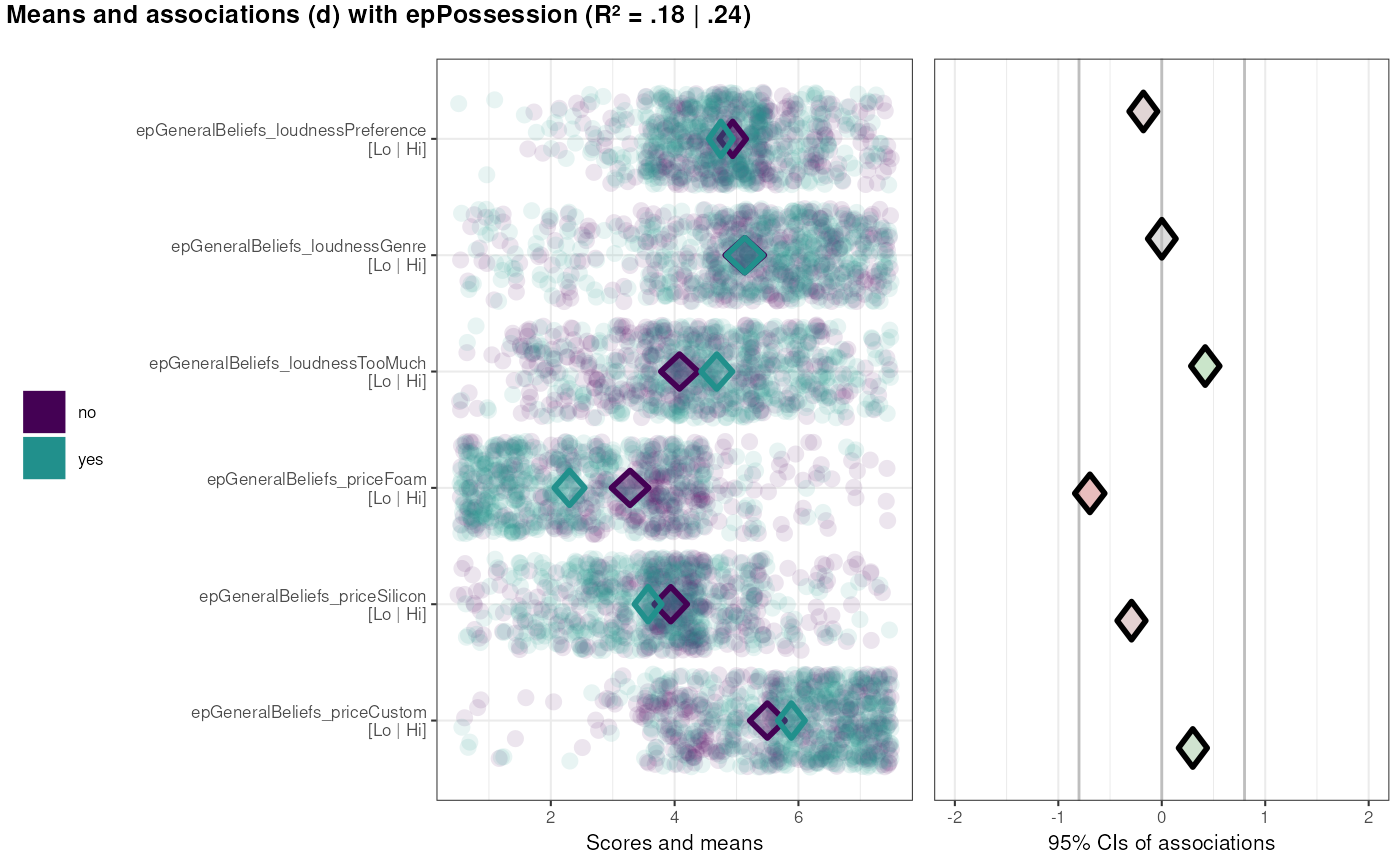This function generates a high-level plot consisting of several diamond plots. This function is useful for estimating the relative relevance of a set of determinants of, for example, behavior. The plot in the left hand panel shows each determinant's distribution with a diamond representing the confidence interval. The right hand plot shows the determinants' associations to one or more 'target' variables, such as behavior or determinants of behavior.

CIBER(
data,
determinants,
targets,
conf.level = list(means = 0.9999, associations = 0.95),
subQuestions = NULL,
leftAnchors = rep("Lo", length(determinants)),
rightAnchors = rep("Hi", length(determinants)),
outputFile = NULL,
outputWidth = NULL,
outputHeight = NULL,
outputUnits = "in",
outputParams = list(),
orderBy = NULL,
decreasing = NULL,
numberSubQuestions = FALSE,
generateColors = list(means = c("red", "blue", "green"), associations = c("red",
"grey", "green")),
strokeColors = viridis::viridis(length(targets)),
vLines = c(-0.5, 0, 0.5),
vLineColors = "grey",
titlePrefix = "Means and associations (r) with",
titleVarLabels = NULL,
titleSuffix = "",
fullColorRange = NULL,
associationsAlpha = 0.5,
returnPlotOnly = TRUE,
drawPlot = TRUE,
jitterWidth = 0.45,
baseSize = 0.8,
dotSize = 2.5 * baseSize,
baseFontSize = 10 * baseSize,
theme = ggplot2::theme_bw(base_size = baseFontSize),
xbreaks = NULL,
rsq = TRUE,
...
)

binaryCIBER(
data,
determinants,
targets,
conf.level = list(means = 0.9999, associations = 0.95),
subQuestions = NULL,
leftAnchors = rep("Lo", length(determinants)),
rightAnchors = rep("Hi", length(determinants)),
outputFile = NULL,
outputWidth = NULL,
outputHeight = NULL,
outputUnits = "in",
outputParams = list(),
orderBy = NULL,
decreasing = NULL,
numberSubQuestions = FALSE,
comparisonColors = viridis::viridis(2, end = 0.5),
categoryLabels = NULL,
generateColors = list(means = c("red", "blue", "green"), associations = c("red",
"grey", "green")),
strokeColors = viridis::viridis(length(targets)),
vLines = c(-0.8, 0, 0.8),
vLineColors = "grey",
titlePrefix = "Means and associations (d) with",
titleVarLabels = NULL,
titleSuffix = "",
fullColorRange = NULL,
associationsAlpha = 0.5,
returnPlotOnly = TRUE,
drawPlot = TRUE,
baseSize = 0.8,
dotSize = 2.5 * baseSize,
baseFontSize = 10 * baseSize,
theme = ggplot2::theme_bw(base_size = baseFontSize),
xbreaks = NULL,
...
)

detStructCIBER(
determinantStructure,
data,
conf.level = list(means = 0.9999, associations = 0.95),
subQuestions = NULL,
leftAnchors = rep("Lo", length(determinants)),
rightAnchors = rep("Hi", length(determinants)),
orderBy = 1,
decreasing = NULL,
generateColors = list(means = c("red", "blue", "green"), associations = c("red",
"grey", "green")),
strokeColors = NULL,
titlePrefix = "Means and associations with",
titleVarLabels = NULL,
titleSuffix = "",
fullColorRange = NULL,
associationsAlpha = 0.5,
baseSize = 0.8,
dotSize = 2.5 * baseSize,
baseFontSize = 10 * baseSize,
theme = ggplot2::theme_bw(base_size = baseFontSize),
...
)

## Arguments

data

The dataframe containing the variables.

determinants

The 'determinants': the predictors (or 'covariates') of the target variables(s) (or 'criteria').

targets

The 'targets' or 'criteria' variables: the variables predicted by the determinants.

conf.level

The confidence levels for the confidence intervals: has to be a named list with two elements: means and associations, specifying the desired confidence levels for the means and associations, respectively. The confidence level for the associations is also used for the intervals for the proportions of explained variance.

subQuestions

The subquestions used to measure each determinants. This can also be used to provide pretty names for the variables if the determinants were not measured by one question each. Must have the same length as determinants.

leftAnchors

The anchors to display on the left side of the left hand panel. If the determinants were measured with one variable each, this can be used to show the anchors that were used for the respective scales. Must have the same length as determinants.

rightAnchors

The anchors to display on the left side of the left hand panel. If the determinants were measured with one variable each, this can be used to show the anchors that were used for the respective scales. Must have the same length as determinants.

outputFile

The file to write the output to (the plot is not stored to disk if NULL). The extension can be specified to change the file type.

outputWidth, outputHeight, outputUnits

The width, height, and units for the output file.

outputParams

More advanced parameters for the output file. This can be used to pass arguments to ggplot2::ggsave(), such as passing outputParams=list(type="cairo-png") to use anti-aliasing when saving a PNG file.

orderBy

Whether to sort the determinants. Set to NULL to not sort at all; specify the name or index of one of the targets to sort by the point estimates of the associations with that target variable. Use decreasing to determine whether to sort in ascending or descending order. For convenience, if orderBy is not NULL, but decreasing is, the determinants are sorted in descending (decreasing) order.

decreasing

Whether to sort the determinants. Specify NULL to not sort at all, TRUE to sort in descending order, and FALSE to sort in ascending order. If decreasing is nor NULL, but orderBy is NULL, the determinants are sorted by their means. For convenience, if orderBy is not NULL, but decreasing is, the determinants are sorted in descending (decreasing) order.

numberSubQuestions

Whether or not to number the subquestions. If they are numbered, they are numbered from the top to the bottom.

generateColors

The colors to use to generate the gradients for coloring the diamonds representing the confidence intervals. Has to be a named list with two elements: means and associations, specifying the desired colors for the means and associations, respectively.

strokeColors

The palette to use to color the stroke of the confidence intervals for the associations between the determinants and the targets. Successive colors from this palette are used for the targets.

vLines, vLineColors

In the association plot, vertical lines can be plotted to facilitate interpretation. Specify their locations and colors here, or set one or both to NULL to eliminate them.

titlePrefix

Text to add before the list of target names and the proportions of explained variance for each target. This plot title also serves as legend to indicate which target 'gets' which each color.

titleVarLabels

Optionally, variable labels to use in the plot title. Has to be the exact same length as targets.

titleSuffix

Text to add after the list of target names and the proportions of explained variance for each target.

fullColorRange

If colors are specified, this can be used to specify which values, for the determinant confidence intervals in the left hand panel, are the minimum and maximum. This is useful if those scores are not actually in the data (e.g. for extremely skewed distributions). If NULL, the range of all individual scores on the determinants is used. For the associations, c(-1, 1) is always used as fullColorRange.

associationsAlpha

The alpha level (transparency) of the confidence interval diamonds in the right hand plot. Value between 0 and 1, where 0 signifies complete transparency (i.e. invisibility) and 1 signifies complete 'opaqueness'.

returnPlotOnly

Whether to return the entire object that is generated (including all intermediate objects) or only the plot.

drawPlot

Whether the draw the plot, or only return it.

jitterWidth

How much to jitter the data points in the left hand plot.

baseSize

This can be used to efficiently change the size of most plot elements.

dotSize

This is the size of the points used to show the individual data points in the left hand plot.

baseFontSize

This can be used to set the font size separately from the baseSize.

theme

This is the theme that is used for the plots.

xbreaks

Which breaks to use on the X axis (can be useful to override ggplot2's defaults).

rsq

Whether to compute the R squared values.

...

These arguments are passed on to biAxisDiamondPlot (for the left panel) and diamondPlot (for the right panel). Note that all argument are passed to both those functions.

comparisonColors

Colors to use for the two groups in a binary CIBER plot with one (dichotomous) target.

categoryLabels

Labels for the two values of the target.

determinantStructure

When using detStructCIBER, the determinant structure as generated by determinantStructure is included here. determinants, targets, subQuestions, leftAnchors, and rightAnchors are then read from the determinantStructure object. In other words: once a determinantStructure has been generated, only dat and determinantStructure have to be provided as argument to generate a CIBER diamond plot.

## Value

Depending on the value of returnPlotOnly, either the plot only (a gtable object) or an object containing most objects created along the way (in which case the plot is stored in $output$plot).

The plot has width and height attributes which can be used when saving the plot.

## Details

Details are explained in Crutzen & Peters (2017).

determinantStructure

## Examples

### This example uses the determinant study Party Panel 17.1;
data(BBC_pp17.1);
behaviorchange::CIBER(data=BBC_pp17.1,
determinants=c('epw_AttExpect_hearingDamage',
'epw_AttExpect_highTone',
'epw_AttExpect_musicVolume',
'epw_AttExpect_musicFidelity',
'epw_AttExpect_loudConversation',
'epw_AttExpect_musicFocus',
'epw_AttExpect_musicEnjoy'),
targets=c('epw_attitude'));### With a binary target
data(BBC_pp17.1);
behaviorchange::binaryCIBER(data=BBC_pp17.1,
determinants=c('epGeneralBeliefs_loudnessPreference',
'epGeneralBeliefs_loudnessGenre',
'epGeneralBeliefs_loudnessTooMuch',
'epGeneralBeliefs_priceFoam',
'epGeneralBeliefs_priceSilicon',
'epGeneralBeliefs_priceCustom'),
targets=c('epPossession'),
categoryLabels = c('no',
'yes'));